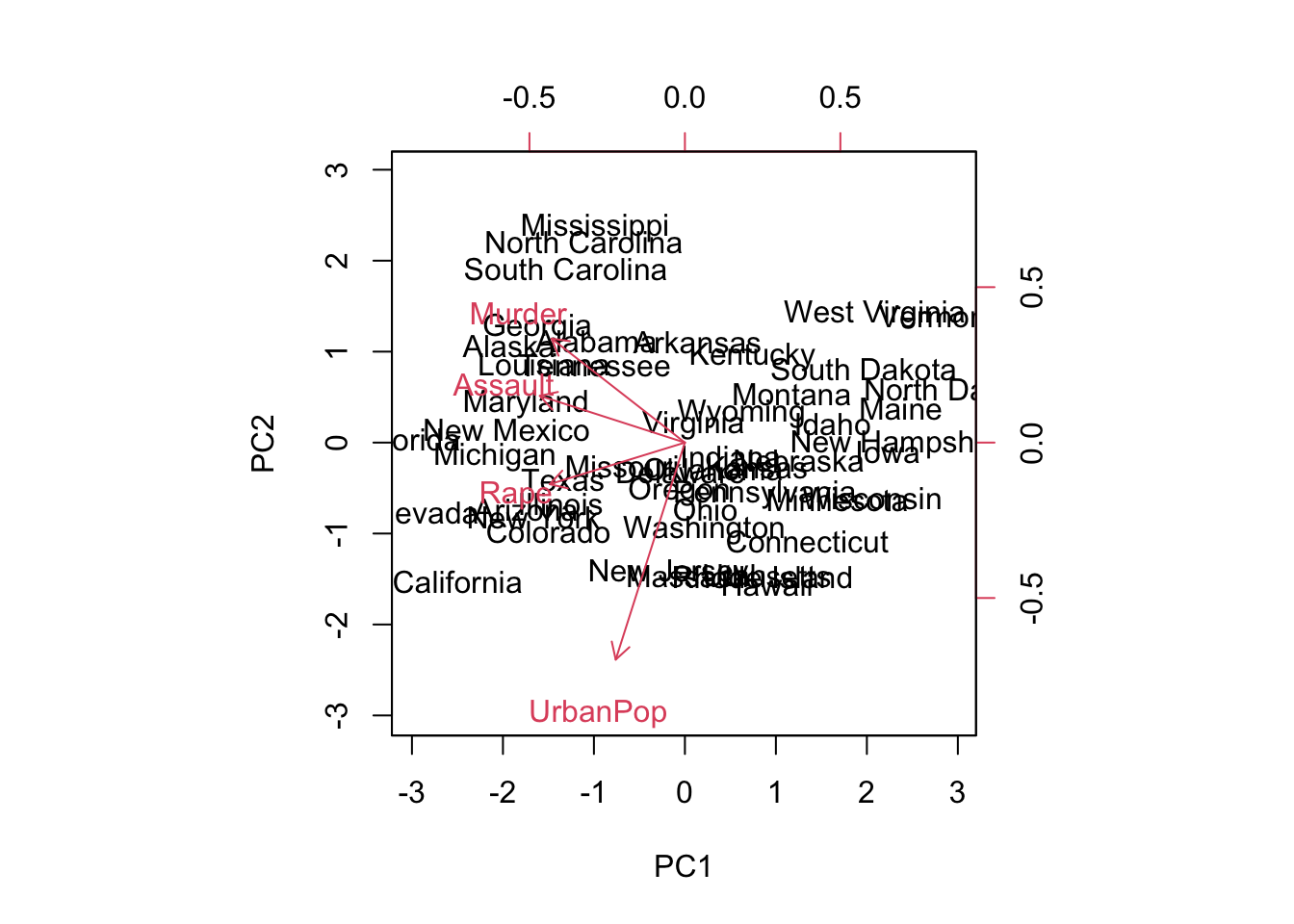ISLR Home

p401

We perform PCA on the USArrests data set, which is part of the base R package. The rows of the data set contain the 50 states, in alphabetical order.

``````states=row.names(USArrests)
#states
names(USArrests)``````
``##  "Murder"   "Assault"  "UrbanPop" "Rape"``

Apply the mean to each column

``apply(USArrests, 2, mean)``
``````##   Murder  Assault UrbanPop     Rape
##    7.788  170.760   65.540   21.232``````

Apply the variance to each column

``apply(USArrests, 2, var)``
``````##     Murder    Assault   UrbanPop       Rape
##   18.97047 6945.16571  209.51878   87.72916``````

## Perform principal components analysis

Use the prcomp() function.

By default, the prcomp() function centers the variables to have mean zero. By using the option scale=TRUE, we scale the variables to have standard deviation one.

``````pr.out=prcomp(USArrests, scale=TRUE)
summary(pr.out)``````
``````## Importance of components:
##                           PC1    PC2     PC3     PC4
## Standard deviation     1.5749 0.9949 0.59713 0.41645
## Proportion of Variance 0.6201 0.2474 0.08914 0.04336
## Cumulative Proportion  0.6201 0.8675 0.95664 1.00000``````
``pr.out\$center``
``````##   Murder  Assault UrbanPop     Rape
##    7.788  170.760   65.540   21.232``````
``pr.out\$scale``
``````##    Murder   Assault  UrbanPop      Rape
##  4.355510 83.337661 14.474763  9.366385``````
``names(pr.out)``
``##  "sdev"     "rotation" "center"   "scale"    "x"``

``pr.out\$rotation``
``````##                 PC1        PC2        PC3         PC4
## Murder   -0.5358995  0.4181809 -0.3412327  0.64922780
## Assault  -0.5831836  0.1879856 -0.2681484 -0.74340748
## UrbanPop -0.2781909 -0.8728062 -0.3780158  0.13387773
## Rape     -0.5434321 -0.1673186  0.8177779  0.08902432``````

There are four distinct principal components. In general min(n - 1, p) informative principal components in a data set with n observations and p variables.

``dim(pr.out\$x)``
``##  50  4``

## Plot first two principal components

``biplot(pr.out, scale=0)``The scale=0 argument to biplot() ensures that the arrows are scaled to represent the loadings; other values for scale give slightly different biplots with different interpretations.

## Reproduce Figure 10.1

``````pr.out\$rotation=-pr.out\$rotation
pr.out\$x=-pr.out\$x
biplot(pr.out, scale=0)``````